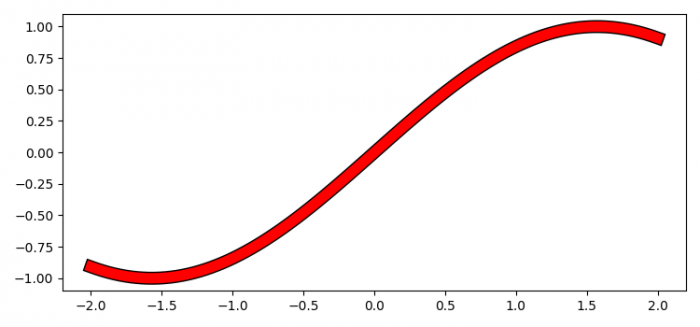# Can I give a border to a line in Matplotlib plot function?

To give a border to a line in matplotlib plot function, we can call plot() function twice with varying line width.

## Steps

• Set the figure size and adjust the padding between and around the subplots.
• Create x and y data points using numpy.
• Plot x and y data points where line width=10 and color=black.
• Again plot x and y points where line width=8 and color=red.
• To display the figure, use show() method.

## Example

import numpy as np
from matplotlib import pyplot as plt
plt.rcParams["figure.figsize"] = [7.50, 3.50]
plt.rcParams["figure.autolayout"] = True
x = np.linspace(-2, 2, 100)
y = np.sin(x)
plt.plot(x, y, c='black', lw=10)
plt.plot(x, y, c='red', lw=8)
plt.show()

## Output